Function Repository Resource:

# EnlargeBoundingBox

Increase a bounding box by a fractional amount

Contributed by: Taliesin Beynon (Wolfram Research)
 ResourceFunction["EnlargeBoundingBox"][{{l,r},{b,t}},frac] enlarges the given bounding box by the fraction frac of its width and height. ResourceFunction["EnlargeBoundingBox"][{{l,r},{b,t}},{{dl,dr},{db,dt}}] enlarges the given bounding box by the given amount on each side.

## Details and Options

A zero fraction gives the graphics with a {{l,r},{b,t}} bounding box.

## Examples

### Basic Examples (3)

Basic usage:

 In:=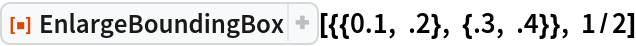Out=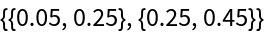Feed in Graphics objects:

 In:=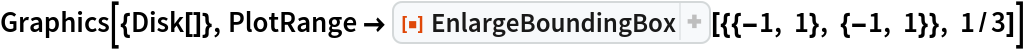Out=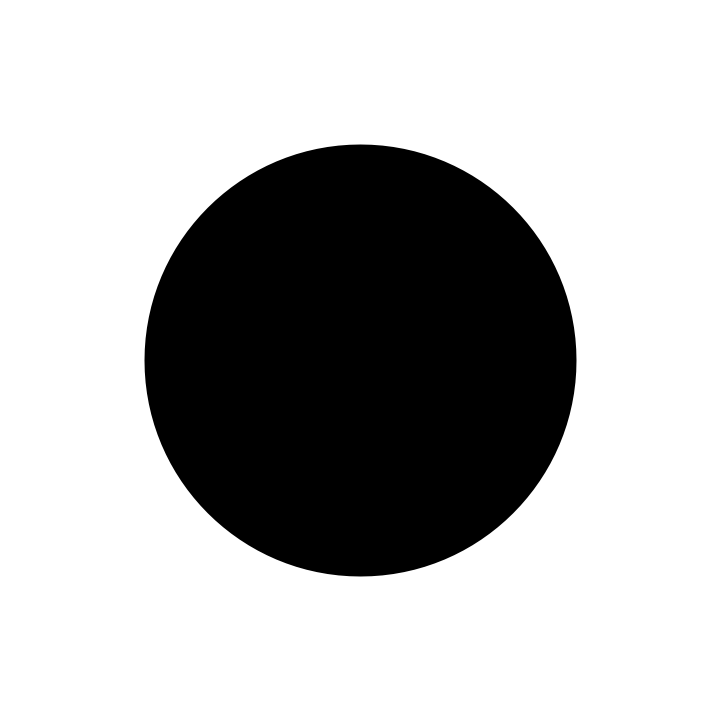Adjust each side, increasing the extent as we go from left to right and then bottom to top:

 In:=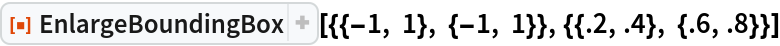Out=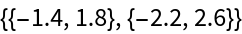In:=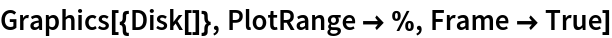Out=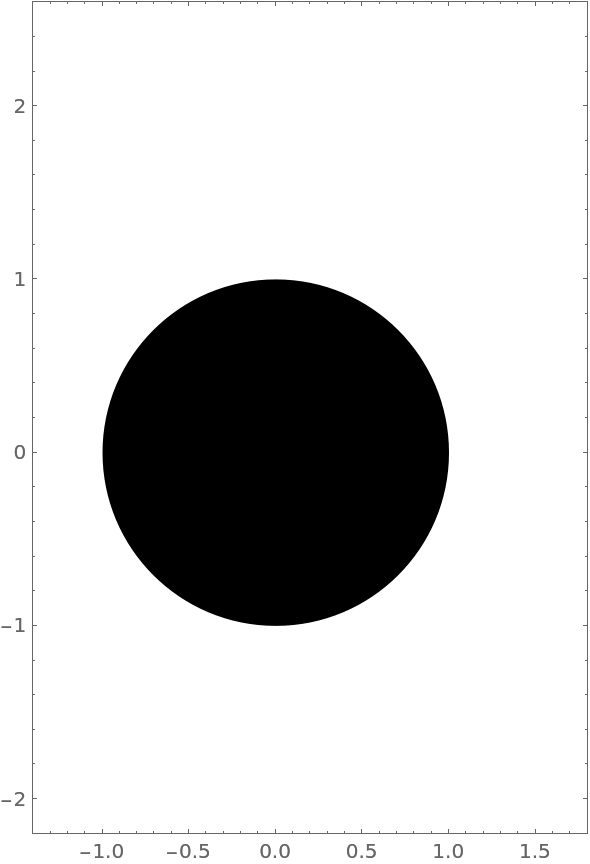### Scope (1)

EnlargeBoundingBox also works on 3D graphics:

 In:=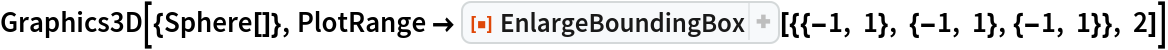Out=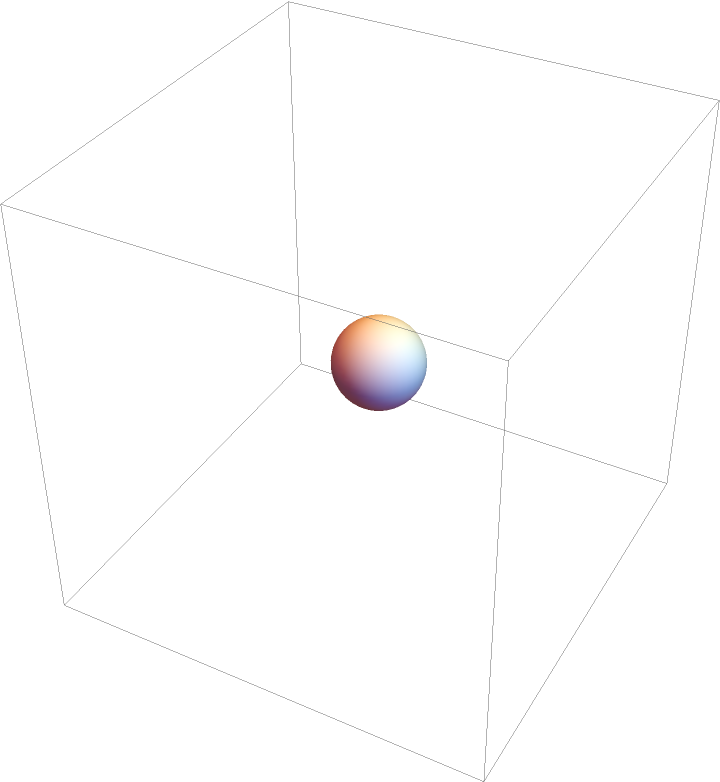### Applications (1)

Create a zoom effect:

 In:=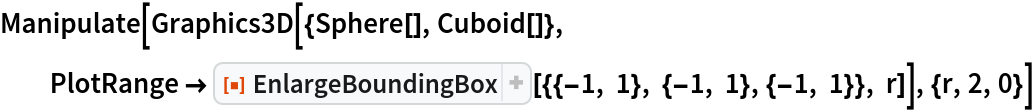Out=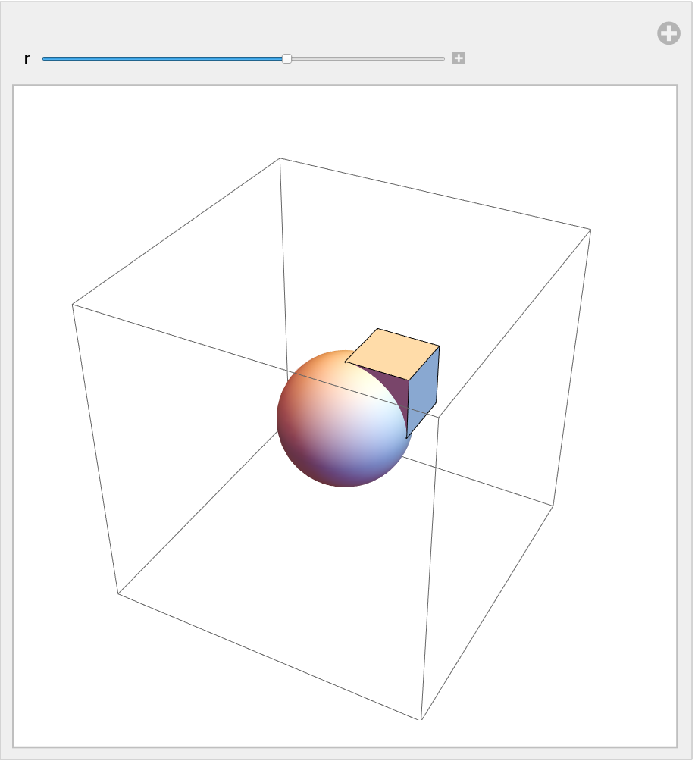Enrique Zeleny

## Version History

• 1.0.0 – 29 June 2020